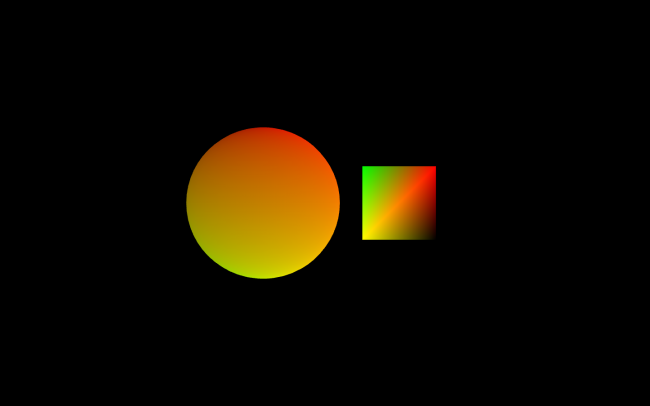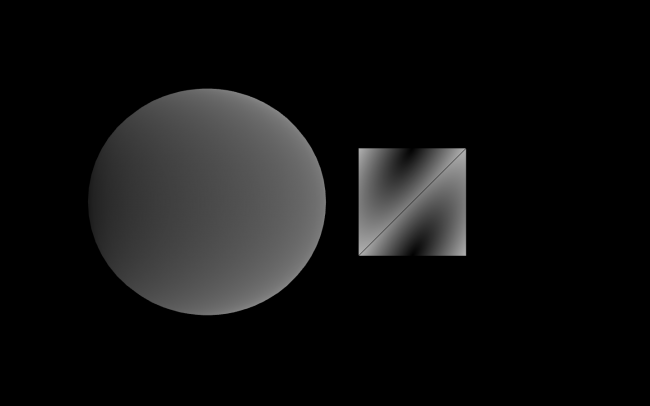#### Howdy, Stranger!

We are about to switch to a new forum software. Until then we have removed the registration on this forum.

# texCoord problem

hello! its me again. im in a hardest process of learning GLSL. As i can see, exist many types of sintaxis and versions for writing a shader program and its a little bit confuse writing a shader for Processing. In this time, i`ve two questions.

1) im reading OpenGl 4.0 Shading Lenguaje Cookbook. One of the first examples, use it a block of uniforms. How do i set a uniform block in Processing? cos if i set one by one it doesnt work.

``````uniform BlobSettings {
vec4 InnerColor;
vec4 OuterColor;
};
``````

In processing:

``````   sh.set("RadiusOuter", 10.0 );
sh.set("InnerColor", c);
sh.set("OuterColor", b);
``````

2) Following this light house tutorial http://www.lighthouse3d.com/tutorials/glsl-tutorial/texture-coordinates/ im trying to do the example of textCoord, and it doesnt work... here the code:

``````PShader sh;
PShape t;

import peasy.*;
import peasy.org.apache.commons.math.*;
import peasy.org.apache.commons.math.*;
PeasyCam cam;

void setup(){
cam = new PeasyCam(this, 800);
size(500,500, P3D);
t = trian();
}

void draw(){
background(0);
shape(t);

}

PShape trian (){

PShape t = createShape();
// t.fill(random(255),0,0);
t.vertex(-200, -200);
// t.fill(0,255,0);
t.vertex(-200, 200);
// t.fill(0,0,255);
t.vertex(200,-200);
t.vertex(200, 200);
t.endShape();

return t;
}
``````

``````#version 330

uniform mat4 transform;

in vec4 position;
in vec3 color;
in vec2 texCoord;

out vec3 vertColor;
out vec2 TexCoord;

void main(){

TexCoord = texCoord;
vertColor = color;
gl_Position = transform * position;
}
``````

``````#ifdef GL_ES
precision mediump float;
#endif

in vec3 vertColor;
in vec2 TexCoord;

void main() {

gl_FragColor = vec4(TexCoord,1.0,1.0);
}
``````

was suppossed to be this result:

but its only a blue rect. and its becouse TexCoord value is 0 and im lost why... how fragCoord works?

thanks.

1.)
you have to use low level jogl mode. no uniform blocks in processing.

vertex(x, y, z, u, v)

Note: your fragmentShader no version means in processing #version 100 (es) so ifdef GL_ES only needed if you want to target OpenGL ES Version 2.0
https://en.wikibooks.org/wiki/OpenGL_Programming/Modern_OpenGL_Tutorial_03#OpenGL_ES_2_portability
#version 330 so you targeting different versions. And it still work. Thx Java!

their is a compatibility vs core preprocessor directive behind the scenes you can add it if you like for whatever reason
#version 330 compatibility
#version 330 core

https://jogamp.org/jogl/doc/Overview-OpenGL-Evolution-And-JOGL.html

the default processing is GL2ES2

how fragCoord works? google it, their are much greater tutorials and explanation as i got time :)

• edited January 2018

Yes just to be complete:
#version 100
is becoming #version 300 es in OpenGL ES Version 3.0

this old back then (~10years) smartphones coming slowly to regular use. that is why you use ifdef GL_ES with #version 120 and in the newer versions only es But their are still quite few older smartphones around that only support OpenGL ES Version 2.0. So if you programing a simpel Game, and not the next Battelfield Game for Android you want to program in GL2ES2 and check ifdef GL_ES (Not for WEBGL) My smartphone is 5years old, i just use it to make a call, so i got max OpenGL ES Version 3.1

Also note that on the CPU side in JOGL the things are changing too. So it's not only the shader version.

And that is why your question is self explaining, becourse GL2ES2 has no Uniform Blocks.

• update a progress, maybe it help anybody:

(thanks @nabr, im gonna to drive you insane);

Ok, so after hours and hours (think im a little bit stupid) i made a little progress with shaders and processing. I finally could use the texCoord in lightHouse tutorial:processing:

``````PShader sh;
PShape t;

import peasy.*;
import peasy.org.apache.commons.math.*;
import peasy.org.apache.commons.math.*;
float c [] = {0.0, 0.1,1.0, 1.0};
float b [] = {1.0, 0.1,1.0, 1.0};
PeasyCam cam;
float count;
void setup(){
cam = new PeasyCam(this, 500);
// size(500,500, P3D);
fullScreen(P3D);
t = trian();
}

void draw(){
background(0);
// cam.rotateY(0.05);
count +=0.09;
sh.set("u_time", count);
// sh.set("InnerColor", c);
// sh.set("OuterColor", b);
pushMatrix();
scale(100);
shape(t);
noStroke();
popMatrix();
pushMatrix();
translate(-width/4, 0,0);
sphere(200);
popMatrix();
}

PShape trian (){
textureMode(NORMAL);
PShape t = createShape();
t.vertex(-1,-1,0,    0,  1);
t.vertex(-1,1,0,     1,  1);
t.vertex(1,-1,0,     1,  0);
t.vertex(1,1,0,      0,  0);

t.endShape();

return t;
}
``````

# version 150

``````uniform mat4 transform;
in vec4 position;
in vec2 texCoord;
out vec2 TexCoord;

void main(){

TexCoord = texCoord;
vertColor = position.xyz;

gl_Position = transform * position;
}
``````

``````    #ifdef GL_ES
precision mediump float;
#endif

in vec2 TexCoord;

void main() {

gl_FragColor = vec4(TexCoord,0.0, 1.0);

}
``````
• what happens me now is that i cant found the center of the QUAD, im trying following bookofShader algorithm:

``````PShape trian (){
textureMode(NORMAL);
PShape t = createShape();
t.vertex(-1,-1,0,    0,  1);
t.vertex(-1,1,0,     1,  1);
t.vertex(1,-1,0,     1,  0);
t.vertex(1,1,0,      0,  0);

t.endShape();

return t;
}
``````

in fragment:

``````#ifdef GL_ES
precision mediump float;
#endif

in vec2 TexCoord;
//
// uniform  vec4 InnerColor;
// uniform  vec4 OuterColor;
// uniform float u_time;

void main() {

vec2 st = TexCoord;
float toCenter = distance(st, vec2(0.5));

vec3 color = vec3(toCenter);

gl_FragColor = vec4(color, 1.0);
// gl_FragColor = vec4(1.0,1.0,1.0,1.0);
}
``````

result:what im doing wrong? maybe set the UV coordinates in vertex? follow my instint, dont know exactly what that numbers mean. any suggestion?

EDIT:

got it, but still dont get it;

``````PShape trian (){
textureMode(NORMAL);
PShape t = createShape();

t.vertex(-1,-1,0,    1,  1);
t.vertex(-1,1,0,     1,  0);
t.vertex(1,-1,0,     0,  1);
t.vertex(1,1,0,      0,  0);
t.endShape();

return t;
}
``````

change the UV coordinate.

• edited January 2018 Answer ✓

@vjjv Hello
man what can i say, remember learing all this stuff, i look at your code and i know - you skip the very basics and now you are running in some "bugs".
Read a very basic hello triangle: line by line and experiment with it for 1 or two weeks :)
https://learnopengl.com/#!Getting-started/Hello-Triangle ( skip the C++ Code )

``````PShader shdr;
PShape t;
float w,h;
void setup() {
size(640, 360, P2D); // default cam ortho

// P3D
//size(640, 360, P3D); // default cam perspective

w=width*.5f;
h=height*.5f;

noStroke();
new String[]{"#version 150 \n"
+ "in vec4 position;"
+ "in vec2 texCoord;"
+ "uniform mat4 transform;"
+ "out vec2 st;"
+ "void main() {"
+ "st=texCoord.xy;"
+ "gl_Position =vec4(position.xy,0.0,1.);"
// uncomment for P3D
// + "gl_Position =transform*vec4(position.xy*vec2(0.5,-0.5),0.0, /* position.w */ 0.0025 );"
+ "}"
}, new String[]{"#version 150 \n"
+ "smooth in vec2 st;"
+ "out vec4 fragColor;"
+ "void main() {"
+ "fragColor = vec4(st,0.,1.);"
+"}"
});
}
float off =0;

void draw() {

background(127, 255);

// normalized mouse to screen coords 0-1
off=norm( mouseX, 0, width );

t = createShape();
t.beginShape();
t.vertex( 0.0+off, 0.5, 0.0f, 0.0f, 1.0f );   // top right
t.vertex( 0.5f, -0.5f, 0.0f,  1.0f, 1.0f );   // bottom right
t.vertex(-0.5f, -0.50f, 0.0f, 1.0f, 0.0f );   // bottom left
t.endShape();

pushMatrix();
// uncomment for P3D
// translate(w, h, 0.0);
// t.scale(100); // uncomment only if you know what u'r doing
shape(t, 0, 0);
popMatrix();

// reset to default in order to see text

// draw axis coord
textSize(18);
textAlign(CENTER, BOTTOM);

text("0|0", w, h);       // middel
text("0|1", w, 32);      // top
text("1|0", width-32, h);    // right
text("0|-1", w, height); // botton
text("-1|0", 32,h);        // left

//print mouse position
text(""+off,mouseX,mouseY);

}
``````
• off course @narbs. Thanks!